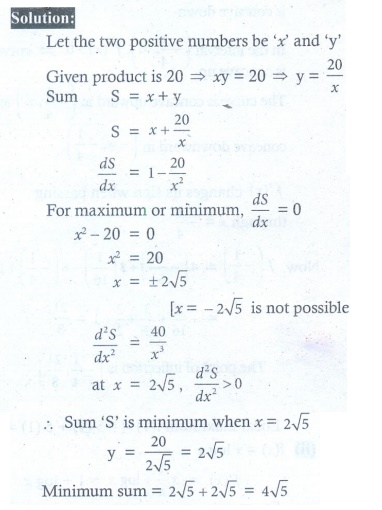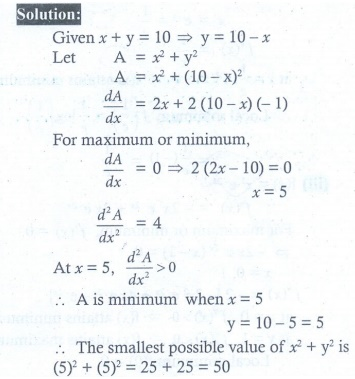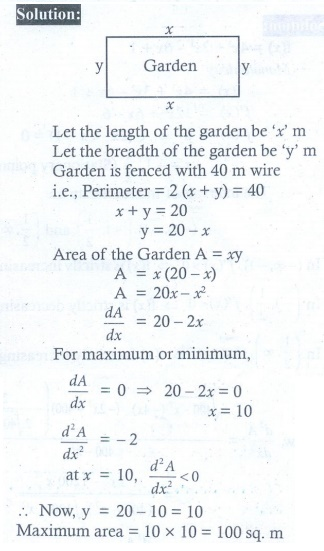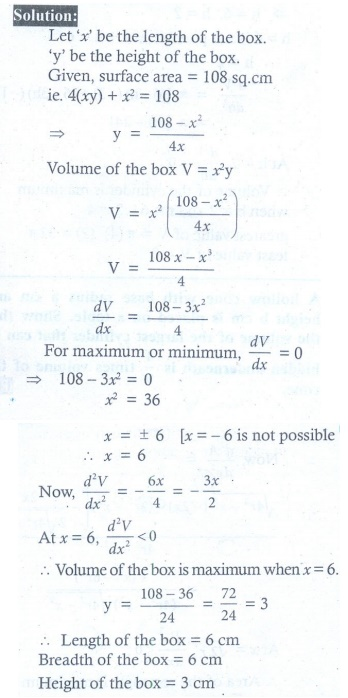Home | | Maths 12th Std | Exercise 7.8: Applications in Optimization

# Exercise 7.8: Applications in Optimization

Maths Book back answers and solution for Exercise questions - Mathematics : Applications of Differential Calculus: Applications in Optimization - Exercise Problem Questions with Answer, Solution

EXERCISE 7.8

1. Find two positive numbers whose sum is 12 and their product is maximum.2. Find two positive numbers whose product is 20 and their sum is minimum.3. Find the smallest possible value of x2 + y2 given that x + y = 10 .4. A garden is to be laid out in a rectangular area and protected by wire fence. What is the largest possible area of the fenced garden with 40 metres of wire.5. A rectangular page is to contain 24 cm2 of print. The margins at the top and bottom of the page are 1.5 cm and the margins at other sides of the page is 1 cm. What should be the dimensions of the page so that the area of the paper used is minimum.6. A farmer plans to fence a rectangular pasture adjacent to a river. The pasture must contain 1,80,000 sq.mtrs in order to provide enough grass for herds. No fencing is needed along the river. What is the length of the minimum needed fencing material?7. Find the dimensions of the rectangle with maximum area that can be inscribed in a circle of radius 10 cm.8. Prove that among all the rectangles of the given perimeter, the square has the maximum area.9. Find the dimensions of the largest rectangle that can be inscribed in a semi circle of radius r cm.10. A manufacturer wants to design an open box having a square base and a surface area of 108 sq.cm. Determine the dimensions of the box for the maximum volume.11. The volume of a cylinder is given by the formula V = ŽĆ r2h . Find the greatest and least values of V if r + h = 6 .12. A hollow cone with base radius a cm and height b cm is placed on a table. Show that the volume of the largest cylinder that can be hidden underneath is 4/9 times volume of the cone.(1) 6ŌĆē,ŌĆē6 ŌĆā

(2) 2ŌłÜ5,2ŌłÜ5

(3) 50

(4) 100m2

(5) 9cm , 6cm

(6) 1200m

(7) 10ŌłÜ2 ,10ŌłÜ2

(9) ŌłÜ2r , r/ŌłÜ2

(10) 6cm , 6cm , 3cm

(11) 32ŽĆ, 0

Tags : Problem Questions with Answer, Solution , 12th Maths : UNIT 7 : Applications of Differential Calculus
Study Material, Lecturing Notes, Assignment, Reference, Wiki description explanation, brief detail
12th Maths : UNIT 7 : Applications of Differential Calculus : Exercise 7.8: Applications in Optimization | Problem Questions with Answer, Solution